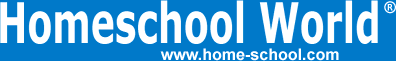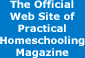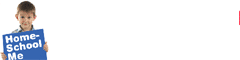Home Curriculum Catalog Articles Contests Events Groups Forum News ContactGetting Started Preschool/K Elementary Middle School High School College & Career Prep Special Needs Group Activities Research FAQs# Elementary Math Part 3

By Bob Hazen
Printed in Practical Homeschooling #14, 1996.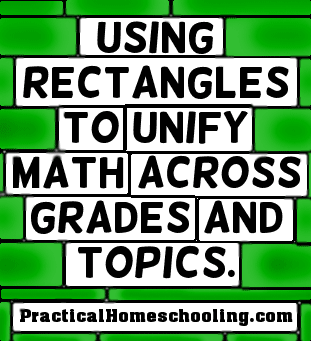Rectangle magic and more!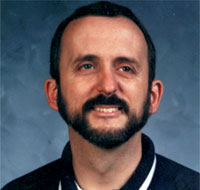One powerful way to broaden and connect elementary math is through the use of effective tools that can be used repeatedly over many grades and across numerous mathematical topics. The rectangle model is one such tool.

The mathematical principle of what is called the "rectangle-area model" uses unit squares in rectangular form as shown in the diagram in Figure 1, where length, width, and area can be examined in distinct but connected ways. The numerous connections from this versatile model come from the different uses that can be related to the simple formula of length x width = area. The formula obviously connects with the operation of multiplication used for a variety of other important math processes.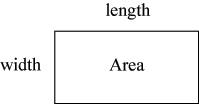Figure 1
Over & Up and the Part-Whole Relationship

To prepare students for the many uses of the rectangle, I have children - even as early as the primary grades (1-3) - simply practice identifying length, width, and area of a rectangular diagram, using rectangles such as those shown in Figure 2.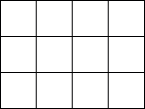Figure 2
For early elementary children, it is no small feat to examine a rectangular grid and identify the length versus the width versus the whole area. The ability to distinguish between the whole and the parts of an object is an important visual, spatial, and geometric skill. This skill is essential to working in real-world situations with diagrams or blueprints and with geometry topics such as perimeter, area, volume, and overlapping shapes. As a secondary math teacher, I can personally testify that even far too many junior high students have not trained their eyes to discern these distinctions.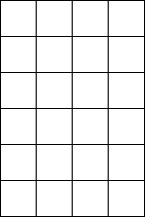Figure 3
I also train students to identify the horizontal "over" amount first and the vertical "up" amount second. This particular order of "over" first, then "up" second, ties in with the skip counting-to-multiplication connection introduced below. Pointing out that the order of "over," then "up" is alphabetical ("o" before "u") helps students remember this. Therefore, a rectangle with an "over" amount of 4 and an "up" amount of 6 would be drawn as shown in figure 3:

Moreover, this rectangle would have a total amount (or area) of 24. Writing this out with symbols (complete with directional arrows to show "over" and "up"), we would have: (4) (6) = 24.

Thus, the first use of the rectangle-area model deals with visual discrimination in a part-whole relationship connected to the important concepts of length, width, and area.

Skip Counting

We also use the rectangle model in skip counting. While skip counting was discussed in the previous article in this series, it bears revisiting from the perspective of the rectangle-area principle. The horizontal rows and vertical columns of rectangles clearly invite skip counting. Numerous household items can be shaped into a rectangular array: screws, cards, coins, or arithmetic unit squares. Children can count how many items are in each horizontal row and then simply skip count the rows. Note that certain items such as pencils or stacks of books are more easily skip counted by simply lumping together into same-size groups rather than being placed in a rectangle array. The rectangle principle is our tool, not our master, so we must use it intelligently, remembering that only many - not all - situations suggest its use. Thus, a second use of the rectangle model connects with the concept-rich process of musical and oral skip counting.

The Transition to Whole Number Multiplication

Skip counting and the rectangle model also set students up for success with multiplication. For example, to count the number of unit squares in the rectangle in Figure 3, a young child (1st-2nd grade) might tend to count the squares one by one. Students who prefer to count by 1's should be encouraged to also skip count the rectangle, confirming to themselves that they arrive, at the same number as when they count by 1's. Skip counting is therefore seen as a shortcut for counting one by one, again with significant help from the use of the rectangle model.

In rectangles such as those in Figure 3, I teach children to skip count the horizontal "over" amount. In this case, students would skip count, "4, 8, 12, 16, 20, 24." After students have mastered skip counting such amounts over a period of time, I have them analyze, this process: if the shortcut for counting by 1's is skip counting, is there, perhaps a shortcut for skip counting itself? Indeed there is . . .

Let's examine our previous counting by 4's - "4, 8, 12, 16, 20, 24." How many times did we count by 4? We counted 4 six times. "4 six times" corresponds to the dimensions of the rectangle: a horizontal "over" distance of 4, and a vertical "up" distance of 6, or (4) (6) = 24.

Therefore, the mental shortcut for skip counting "4, 8, 12, 16, 20, 24" is "count 4 six times," which in written notation is (4) (6) = 24. This notation is very similar to the usual multiplication notation 4 x 6 = 24.

The experiential process for students is thus: counting by 1's; then skip counting as a shortcut for counting by 1's; then the "(over) (up)" notation as a shortcut for skip counting. This process also helps make an easier transition to the regular multiplication "basic fact" form of "4 x 6 = 24."

There are two equally important aspects of basic multiplication facts like "3 x 5 = 15": quick recall and understanding. With this type of principled approach to elementary math, musical skip counting helps ensure the quick recall that 3 x 5 is indeed 15, and the rectangle model combined with skip counting helps students understand what "3 x 5 = 15" really means - counting by 3's five times, or building a rectangle that is 3 "over" and 5 "up," or joining 3's five times. Both quick recall and understanding are needed; neither should be neglected.

To ensure that students understand the process of multiplication, I have students use arithmetic rods to build models of basic multiplication facts: "5 x 8" would mean build a rectangle that is "5 over" and "8 up," and then skip count the total by counting by 5 eight times. "7 x 4" would mean build a rectangle that is "7 over" and "4 up" and then skip count the total by counting by 7 four times. This process has two benefits. First, it builds a conceptual understanding of what multiplication is, because students see what they're doing. Second, it also builds an experiential awareness that simply memorizing that "7 x 4 = 28" is much faster than using manipulatives to build a rectangle that's "7 over" and "4 up."

We have seen that the rectangle model can be used in several ways: part-whole relationships, skip counting, and the transition to whole number multiplication. In Part 2 of this article in the next issue, we will examine several other ways the rectangle can be used: to model other types of multiplication (including 2-digit numbers, fractions, and decimals) as well as division, factoring (including algebra), and percents. The rectangle model's many uses help unify and connect math in powerful ways.Terms of Use   Privacy Policy Copyright ©1993-2023 Home Life, Inc.